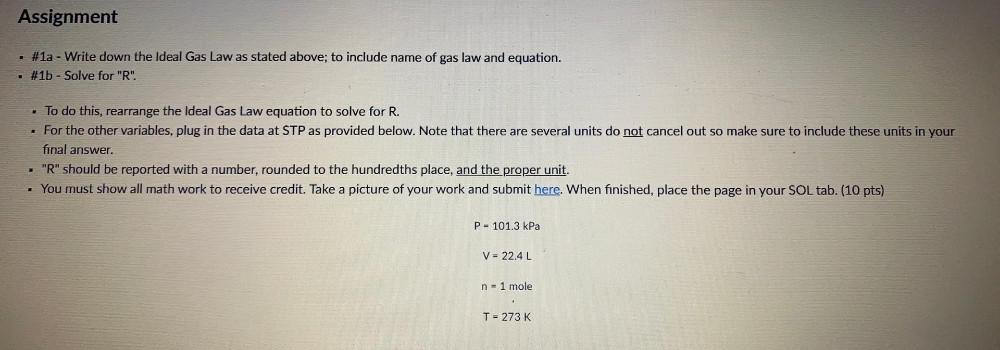Question:

# Assignment #1a - Write down the Ideal Gas Law as stated above; to include name of gas law and equation. #1b - Solve for "R". • TAssignment #1a - Write down the Ideal Gas Law as stated above; to include name of gas law and equation. #1b - Solve for "R". • To do this, rearrange the Ideal Gas Law equation to solve for R. For the other variables, plug in the data at STP as provided below. Note that there are several units do not cancel out so make sure to include these units in your final answer. - "R" should be reported with a number, rounded to the hundredths place, and the proper unit. . You must show all math work to receive credit. Take a picture of your work and submit here. When finished, place the page in your SOL tab. (10 pts) P= 101.3 kPa V = 22.4L n = 1 mole T = 273 K